Select Page

Previous Lesson: Bond Discounting

Next Lesson: Risk and Return

Share Valuation means to find the intrinsic or true value of an investment based only on dividends, cash flow and growth rate for a single company. Shares are ownership in a corporation. It has a certain face value, commonly known as the par value of a share/stock. Ownership of shares is documented by issuance of a stock certificate. A share certificate is a legal document that specifies the amount of shares owned by the shareholder, and other information of the stock, such as the par value, the class of the shares etc.

## Type of Shares

There are two types of Share i.e. Preference Stock and Common / Equity Stocks:

### Preference Share

Pay regular fixed dividend and ownership of the company. Hybrid form of shares because it has equity feature of ownership and debt feature of fixed return. Preference share may be perpetual or redeemable:

#### Preference Share Valuation

Use same equation of Bond valuation: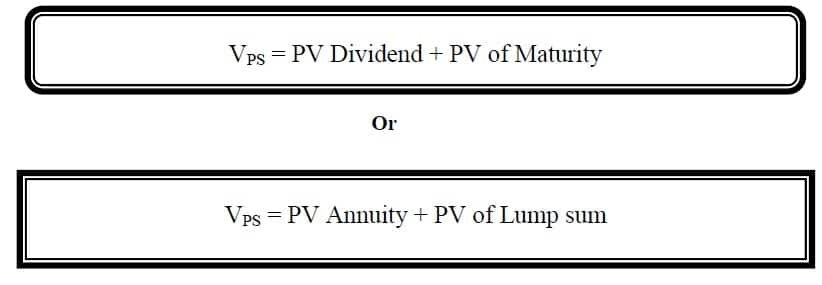#### Formula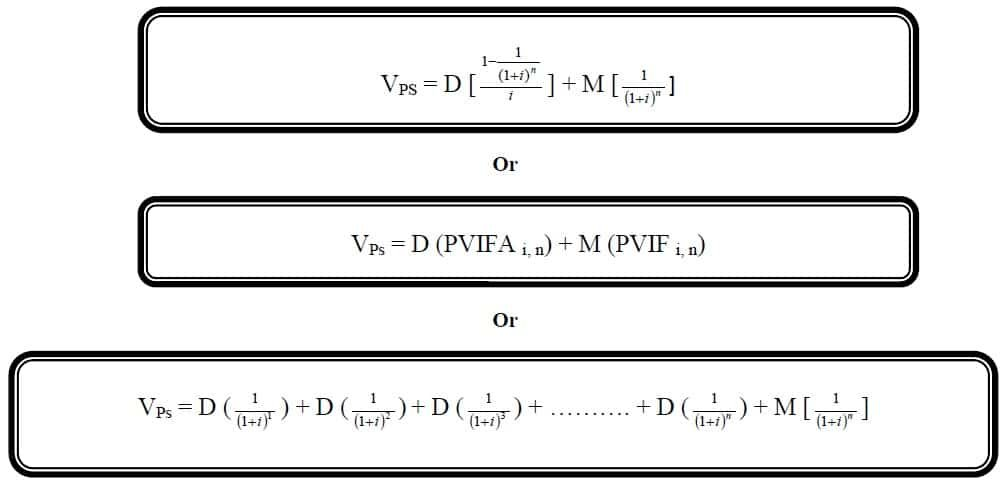### Example 1:

Considered that 8 year, 10 percent dividend preference stock with a par value of Rs. 1,000. The required rate of return on this preference stock is 9 percent. What is the value of this share?### Share Valuation (Redeemable)

Shares are also called stock or common stock. Ownership of the corporation with flexible or floating return. Valuation is based on the same principle of Present Value as bonds, but there are some complications. Uncertainty associated with future cash flows in the forms of dividends and share price. Difficulty in determining an appropriate discount rate.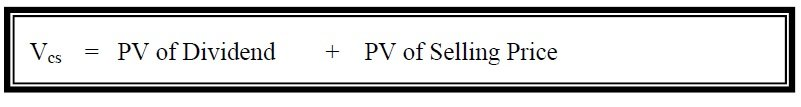#### Single Period Valuation Model

Valuation is depend upon the a year of dividend and after one year sell of shares:### Example 2:

ABC equity share expected to provide a dividend of Rs. 2.00 and market price of Rs. 18 a year hence. What price would it sell for now if investors’ required rate of return is 12 percent?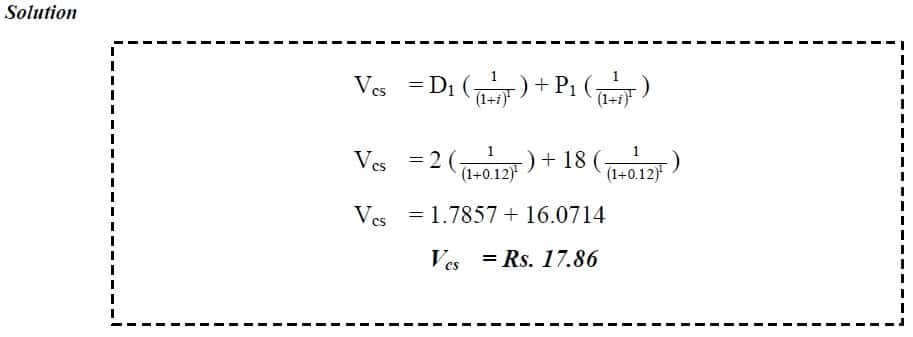#### Multi-Period Valuation Model

Valuation is depend upon more than one year of dividend and after that selling price of shares: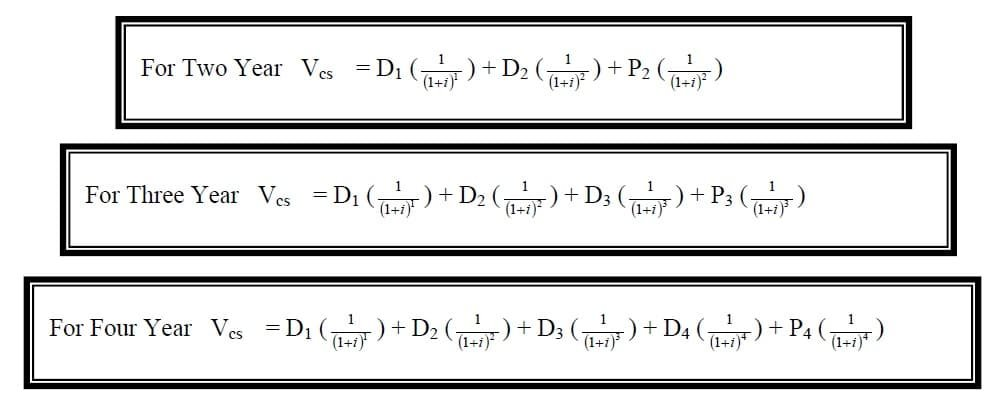### Example 3:

Mr. Ali held 20 share of par value of Rs. 100 each. Dividend for first, second and third year are expected in the amount of Rs. 3, 4 and 5.50 per share respectively and after that share redeemed at face value. Calculate the value of stock if discount rate is 13 %?### Share Valuation (Irredeemable)

There are several methods (models) to assess the value of a stock whose selling price is not known. Valuation are done on the basis of dividend:

#### Dividend Discount Model (DDM)

DDM used compute the intrinsic value of common shares. When time is too long the value of stock depends upon Present value of dividend. Dividend paid annually and first Dividend received one year after the equity share is bought. To compute the value of the stock, all expected future dividend payments are discounted at an appropriate rate to the Present Value:#### Dividend Discount Model (Zero Growth)

Cash flow pattern of zero growth stock is like perpetuity. If we assume that the dividend payments will remain constant then the formula could be written as:### Example 4:

A stock pays a Rs. 12 dividend annually.  If your opportunity cost is 15%, how much is this stock worth to you?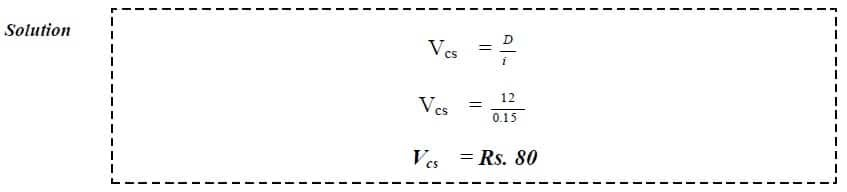#### Dividend Discount Model (Unlimited Constant Growth)

This model assumes that the dividend payments are growing each year at a constant rate of “g” forever: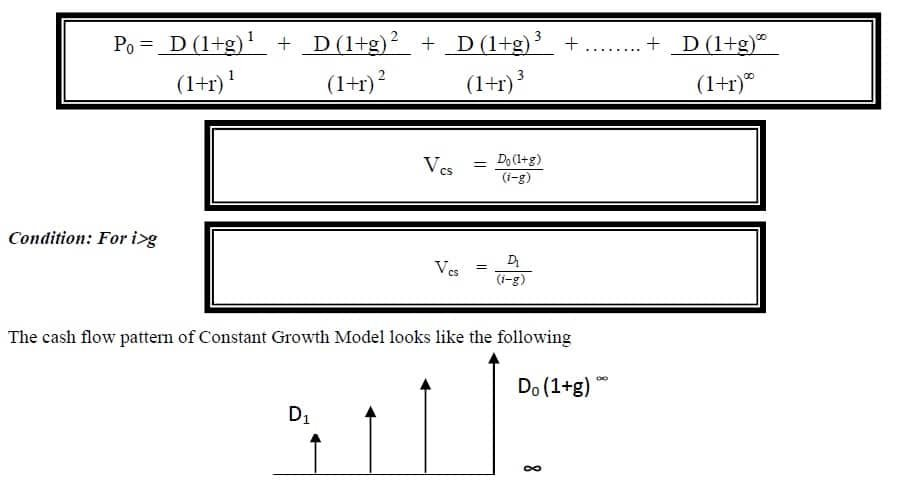### Example 5:

Suppose you want to buy a share of Swiss Farms. Swiss Farms paid a recent dividend at an annual rate of Rs. 2.00 per common share.  After talking to your broker, you expect the dividends to continue to increase at 5% per year, like the past four years.  Your opportunity cost is 12%.  What is this stock worth to you?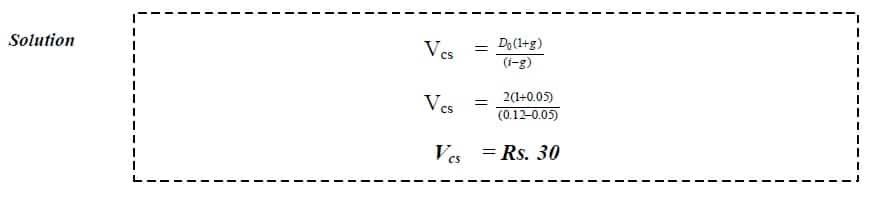#### Dividend Discount Model (Limited Constant Growth)

This model assumes that the dividend payments are growing each year at a constant rate of “g” for limited time### Example 6:

Suppose that Sun Corporation’s dividends this year is Rs. 1.20 per share and that dividends will grow at 10% per year for the next three years, the appropriate discount rate for Corporation’s common stock is 12%.  What is the value of a share?#### Dividend Discount Model (Mixed Growth)

This model assumes that the company and its dividend payments grow much faster than the economy for a certain period at the beginning and then settles to a constant growth rate.

### Example 7:

Suppose that Sun Corporation’s dividends this year is Rs. 1.20 per share and that dividends will grow at 10% per year for the next three years, followed by a 6% annual growth rate.  The appropriate discount rate for Corporation’s common stock is 12%.  What is the value of a share of Sun Corporation common stock?

#### Method 1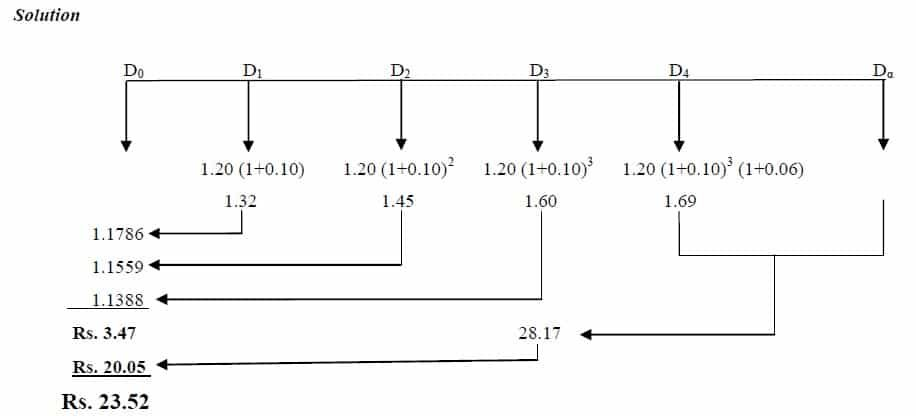#### Method 2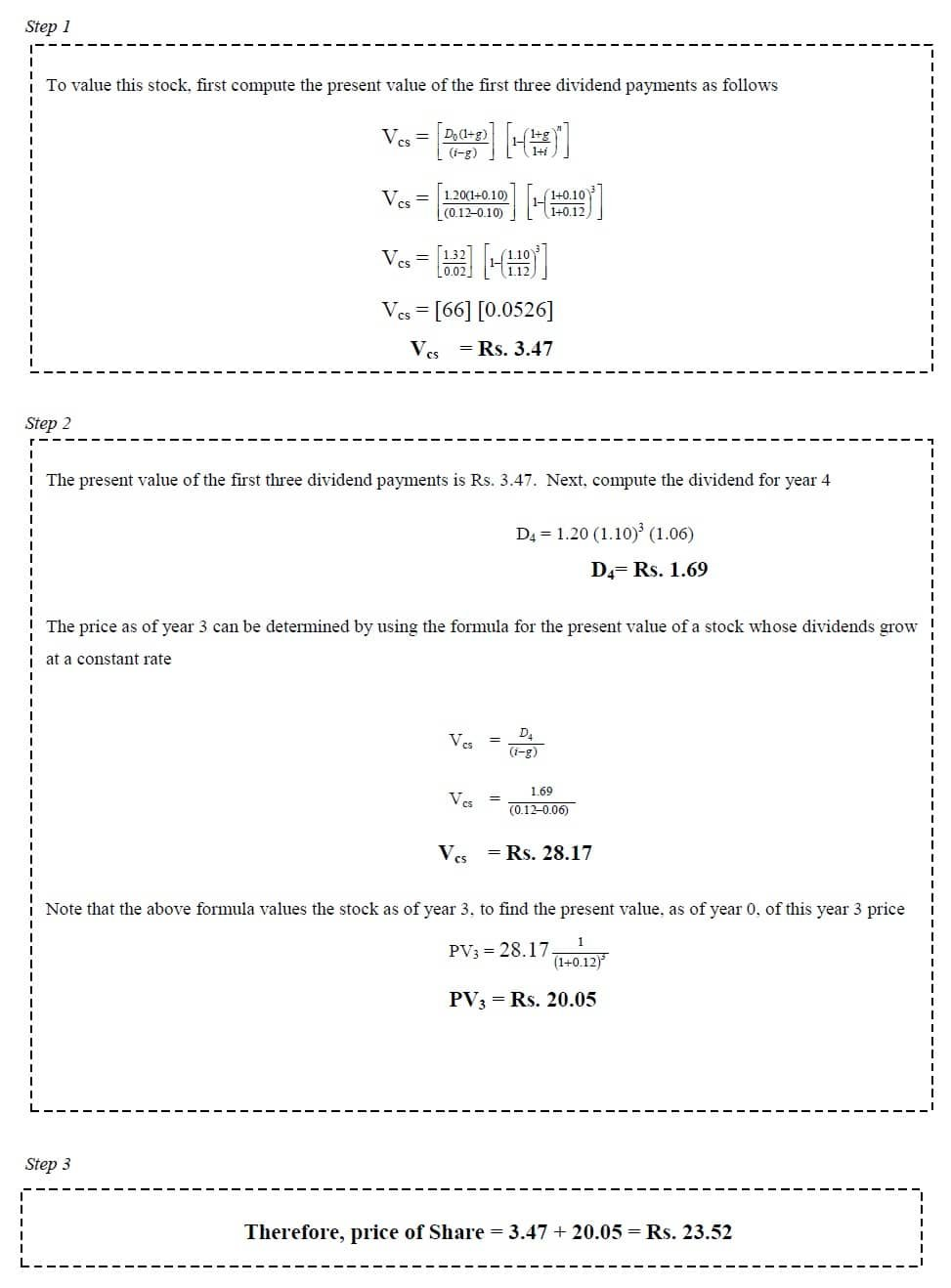#### Method 3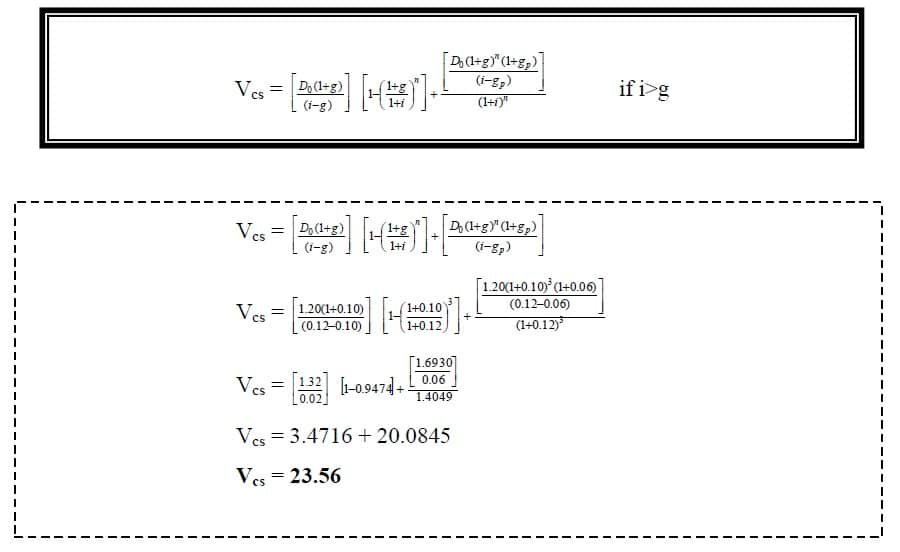>>> Study Share Valuation MCQs.

## Shares Pricing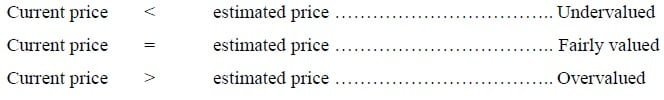The golden rule is ‘buy low, sell high’.

## Expected Rate of Return with Growth### Example 8:

The expected dividend per share of Habib Bank Ltd. is Rs. 5; the dividend is expected to grow at the rate of 6 percent per year. If the price per share now is Rs. 50, what is the expected rate of return?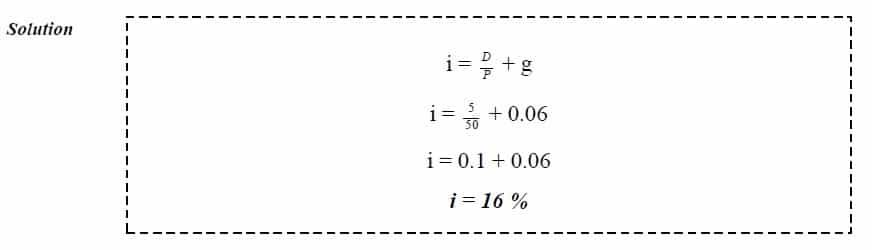>>> Practice Share Valuation Quiz 1

1.